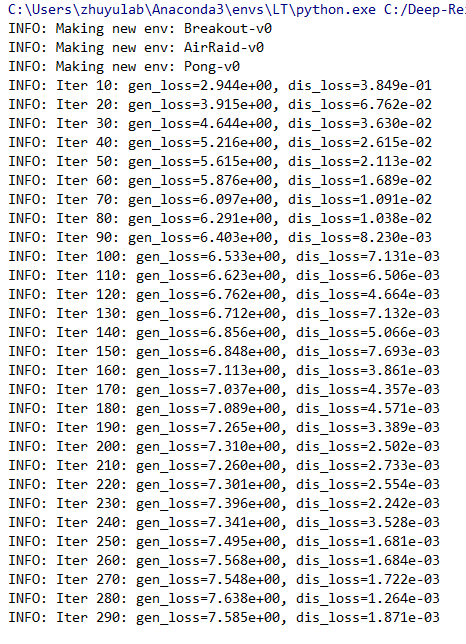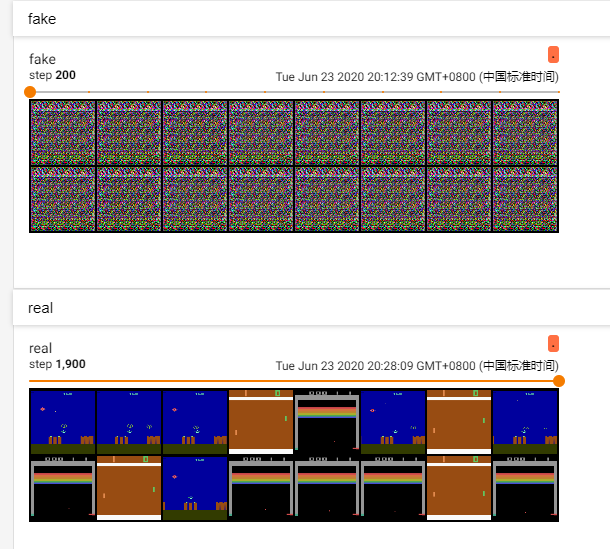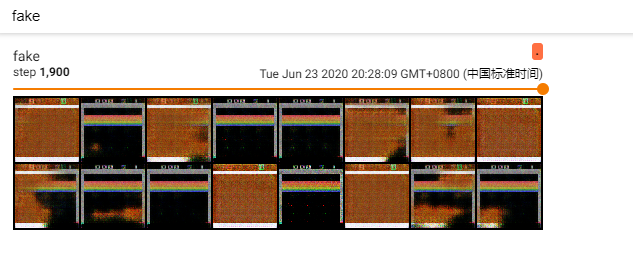# 第三章 实例： 关于Atari游戏的生成对抗网络实现

pip uninstall atari-py
pip install --no-index -f https://github.com/Kojoley/atari-py/releases atari_py


class InputWrapper(gym.ObservationWrapper):
"""
Preprocessing of input numpy array:
1. resize image into predefined size
2. move color channel axis to a first place
"""
def __init__(self, *args):
super(InputWrapper, self).__init__(*args)
assert isinstance(self.observation_space, gym.spaces.Box)
old_space = self.observation_space
self.observation_space = gym.spaces.Box(self.observation(old_space.low), self.observation(old_space.high), dtype=np.float32)

def observation(self, observation):
# resize image
new_obs = cv2.resize(observation, (IMAGE_SIZE, IMAGE_SIZE))
# transform (210, 160, 3) -> (3, 210, 160)
new_obs = np.moveaxis(new_obs, 2, 0)
return new_obs.astype(np.float32)



assert isinstance(self.observation_space, gym.spaces.Box)这是python的assert 用法，
assert expression等价于

if not expression:
raise AssertionError(arguments)


 old_space = self.observation_space
self.observation_space = gym.spaces.Box(self.observation(old_space.low), self.observation(old_space.high), dtype=np.float32)


class Discriminator(nn.Module):
def __init__(self, input_shape):
super(Discriminator, self).__init__()
# this pipe converges image into the single number
self.conv_pipe = nn.Sequential(
nn.Conv2d(in_channels=input_shape, out_channels=DISCR_FILTERS,
nn.ReLU(),
nn.Conv2d(in_channels=DISCR_FILTERS, out_channels=DISCR_FILTERS*2,
nn.BatchNorm2d(DISCR_FILTERS*2),
nn.ReLU(),
nn.Conv2d(in_channels=DISCR_FILTERS * 2, out_channels=DISCR_FILTERS * 4,
nn.BatchNorm2d(DISCR_FILTERS * 4),
nn.ReLU(),
nn.Conv2d(in_channels=DISCR_FILTERS * 4, out_channels=DISCR_FILTERS * 8,
nn.BatchNorm2d(DISCR_FILTERS * 8),
nn.ReLU(),
nn.Conv2d(in_channels=DISCR_FILTERS * 8, out_channels=1,
nn.Sigmoid()
)

def forward(self, x):
conv_out = self.conv_pipe(x)
return conv_out.view(-1, 1).squeeze(dim=1)


net_discr = Discriminator(input_shape=input_shape).to(device)，创建对抗网络实例（鉴别器）, 用print(new_discr)可以打印网络：

print(net_discr)
Discriminator(
(conv_pipe): Sequential(
(0): Conv2d(3, 64, kernel_size=(4, 4), stride=(2, 2), padding=(1, 1))
(1): ReLU()
(2): Conv2d(64, 128, kernel_size=(4, 4), stride=(2, 2), padding=(1, 1))
(3): BatchNorm2d(128, eps=1e-05, momentum=0.1, affine=True, track_running_stats=True)
(4): ReLU()
(5): Conv2d(128, 256, kernel_size=(4, 4), stride=(2, 2), padding=(1, 1))
(6): BatchNorm2d(256, eps=1e-05, momentum=0.1, affine=True, track_running_stats=True)
(7): ReLU()
(8): Conv2d(256, 512, kernel_size=(4, 4), stride=(2, 2), padding=(1, 1))
(9): BatchNorm2d(512, eps=1e-05, momentum=0.1, affine=True, track_running_stats=True)
(10): ReLU()
(11): Conv2d(512, 1, kernel_size=(4, 4), stride=(1, 1))
(12): Sigmoid()
)
)


class Generator(nn.Module):
def __init__(self, output_shape):
super(Generator, self).__init__()
# pipe deconvolves input vector into (3, 64, 64) image
self.pipe = nn.Sequential(
nn.ConvTranspose2d(in_channels=LATENT_VECTOR_SIZE, out_channels=GENER_FILTERS * 8,
nn.BatchNorm2d(GENER_FILTERS * 8),
nn.ReLU(),
nn.ConvTranspose2d(in_channels=GENER_FILTERS * 8, out_channels=GENER_FILTERS * 4,
nn.BatchNorm2d(GENER_FILTERS * 4),
nn.ReLU(),
nn.ConvTranspose2d(in_channels=GENER_FILTERS * 4, out_channels=GENER_FILTERS * 2,
nn.BatchNorm2d(GENER_FILTERS * 2),
nn.ReLU(),
nn.ConvTranspose2d(in_channels=GENER_FILTERS * 2, out_channels=GENER_FILTERS,
nn.BatchNorm2d(GENER_FILTERS),
nn.ReLU(),
nn.ConvTranspose2d(in_channels=GENER_FILTERS, out_channels=output_shape,
nn.Tanh()
)

def forward(self, x):
return self.pipe(x)


def iterate_batches(envs, batch_size=BATCH_SIZE):
batch = [e.reset() for e in envs]
env_gen = iter(lambda: random.choice(envs), None)

while True:
e = next(env_gen)
obs, reward, is_done, _ = e.step(e.action_space.sample())
if np.mean(obs) > 0.01:
batch.append(obs)
if len(batch) == batch_size:
# Normalising input between -1 to 1
batch_np = np.array(batch, dtype=np.float32) * 2.0 / 255.0 - 1.0
yield torch.tensor(batch_np)
batch.clear()
if is_done:
e.reset()



batch = [e.reset() for e in envs]， batch这个列表，是用来保存我们得到的obs数据（我们想要的图片数据其实就是 obs），而一开始， 作者就用e.reset()方法，相当于batch先加入了各个环境的初始obs图片数据——rese方法的返回值就是初始obs。

env_gen = iter(lambda: random.choice(envs), None)，这个用法非常神奇，似乎不是主流的用法， iter(func, None)，通过这样的代码，可以使得func函数变成一个迭代器， 如同后面使用的时候所展示的那样：e = next(env_gen)等价于 e = random.choice(envs)

while True:
yield ....


obs, reward, is_done, _ = e.step(e.action_space.sample())

if len(batch) == batch_size:
# Normalising input between -1 to 1
batch_np = np.array(batch, dtype=np.float32) * 2.0 / 255.0 - 1.0
yield torch.tensor(batch_np)
batch.clear()


if __name__ == "__main__":
parser = argparse.ArgumentParser()
parser.add_argument("--cuda", default=False, action='store_true', help="Enable cuda computation")
args = parser.parse_args()

device = torch.device("cuda" if args.cuda else "cpu")
envs = [InputWrapper(gym.make(name)) for name in ('Breakout-v0', 'AirRaid-v0', 'Pong-v0')]
input_shape = envs.observation_space.shape


net_discr = Discriminator(input_shape=input_shape).to(device)
net_gener = Generator(output_shape=input_shape).to(device)

objective = nn.BCELoss()
gen_optimizer = optim.Adam(params=net_gener.parameters(), lr=LEARNING_RATE, betas=(0.5, 0.999))
dis_optimizer = optim.Adam(params=net_discr.parameters(), lr=LEARNING_RATE, betas=(0.5, 0.999))
writer = SummaryWriter()


gen_losses = []
dis_losses = []
iter_no = 0

true_labels_v = torch.ones(BATCH_SIZE, dtype=torch.float32, device=device)
fake_labels_v = torch.zeros(BATCH_SIZE, dtype=torch.float32, device=device)


for batch_v in iterate_batches(envs):
# generate extra fake samples, input is 4D: batch, filters, x, y
gen_input_v = torch.FloatTensor(BATCH_SIZE, LATENT_VECTOR_SIZE, 1, 1).normal_(0, 1).to(device)
batch_v = batch_v.to(device)
gen_output_v = net_gener(gen_input_v)


for batch_v in iterate_batches(envs):就是将函数iterate_batches看做一个迭代器， 每次循环的时候，到yield关键字处，返回数据。 下一次循环中，再从yield关键字开始， 无限循环。 总之, batch_v 就是这一次循环中，我们用于训练网络的样本数据——真图片。 接下来，我们还要生成假图片。 首先， 随机产生一个向量gen_input_v = torch.FloatTensor(BATCH_SIZE, LATENT_VECTOR_SIZE, 1, 1).normal_(0, 1).to(device), 再通过生成网络, gen_output_v = net_gener(gen_input_v)，按我们的设想，这输出的gen_output_v生成网络产生的图片， 即假图片。 它们和刚刚获取的真图片一起，组成训练数据。

dis_optimizer.zero_grad()
dis_output_true_v = net_discr(batch_v)
dis_output_fake_v = net_discr(gen_output_v.detach())
dis_loss = objective(dis_output_true_v, true_labels_v) + objective(dis_output_fake_v, fake_labels_v)
dis_loss.backward()
dis_optimizer.step()
dis_losses.append(dis_loss.item())


dis_output_true_v = net_discr(batch_v)dis_output_fake_v = net_discr(gen_output_v.detach())分别将 真图片 和假图片， 输入到对抗网络中，进行判别。显然， 损失函数值也就是两者之和了。 objective(dis_output_true_v, true_labels_v)就是对输出和标签求交叉熵。 接下来， 使用backward()方法， 进行计算图中(即神经网络中）所有优化变量的梯度求取， 然后使用优化器的step()方法，对变量进行优化。 最后，将损失值存储。 值得一提的是这里用到了.detach()方法， 该方法返回一个新的变量，但仍指向原来的空间， 且 requires_grad属性默认为False。

gen_optimizer.zero_grad()
dis_output_v = net_discr(gen_output_v)
gen_loss_v = objective(dis_output_v, true_labels_v)
gen_loss_v.backward()
gen_optimizer.step()
gen_losses.append(gen_loss_v.item())


iter_no += 1
if iter_no % REPORT_EVERY_ITER == 0:
log.info("Iter %d: gen_loss=%.3e, dis_loss=%.3e", iter_no, np.mean(gen_losses), np.mean(dis_losses))
gen_losses = []
dis_losses = []
if iter_no % SAVE_IMAGE_EVERY_ITER == 0:
`2000次左右开始就颇具雏形了。tensorboard的用法在上一节中讲述了。

05-11468

#### 【GAN】使用GAN进行mnist数据集中手写图片的生成

01-20962

#### gym atari游戏的环境设置问题：Breakout-v0, Breakout-v4, BreakoutNoFrameskip-v4和BreakoutDeterministic-v4的区别02-22458

#### 强化学习 ---baselines项目之 Atari游戏的网络结构解析

03-171432

#### 增强学习DNQ算法实现Breakout Game（OpenAI/gym +TensorFlow）Ubuntu 16.04

10-053万+

#### 深度强化学习1——强化学习到深度强化学习

08-168458

#### 斯坦福CS231n 2017春季课程全公开，视频+PPT+英文字幕

04-26121

#### 论文阅读笔记 | 用深度强化学习玩Atari

05-271万+

#### 斯坦福大学的 CS231n（全称：面向视觉识别的卷积神经网络）

10-242万+

#### 强化学习（一）

03-051766

#### 图强化学习（结构生成）©️2020 CSDN 皮肤主题: 大白 设计师: CSDN官方博客点击重新获取扫码支付1.余额是钱包充值的虚拟货币，按照1:1的比例进行支付金额的抵扣。
2.余额无法直接购买下载，可以购买VIP、C币套餐、付费专栏及课程。余额充值